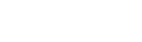# Data Analysis [Part 2 of 5] – Two Variable Data Table in Excel

This is the second article of the five part series on Data Analysis in Excel. In this section, I will show you how to use Two Variable Data Table in Excel.

Other articles in this series:

Watch Video – Two-variable Data Table in Excel

Two-variable data table is best suited in situations when you want to see how the final result changes when two of the input variables change simultaneously (as against One Variable Data Table where only one of the input variable changes).

If you want to analyze data when more than 2 variables change, scenario manager is the way to go.

#### When to use Two Variable Data Table in Excel

Suppose you have a data set as shown below:In the above data set, we have the Loan Amount, Interest Rate, and Number of Monthly payments. Based on these 3 input variables, Monthly Payment is calculated (it is in red as it is an outflow of money). The following formula is used to calculate the Monthly Payment:

`=PMT(B2/12,B3,B1)`

Now you may want to do an analysis to see what should be the ideal combination of Loan Amount and Number of Monthly Payment to suit your requirement. For example, you may want to keep the Monthly Payment at \$500 or less, and analyze what Loan Amount and Tenure combination can give you this.

In such a situation, a two variable data table should be used.

#### Setting up Two Variable Data Table in Excel

Here are the steps to set up a Two variable data table in Excel:

• In a column, have all the different values that you want to test for Number of Monthly Payments. In this example, we are testing for 72, 84, 96… 240. At the same time, have the different loan amount values in the row just above the column values (beginning one cell to the right) as shown in the pic below.• Type =B4 in cell D1, which is one row above the values in the column. This is a construct that needs to be followed when you work with two variable data table. Also, make sure that the value in cell D1 is dependent on both the variables (Number of Monthly Payments and Loan amount). It won’t work if you manually enter the value in cell D1.
In this case, cell D1 refers to cell B4, which has a value calculated using a formula which uses cells B1, B2 and B3.• Now the data is all set to be used for a two variable data table calculation.
• Select the data (D1:J16). Go to Data Tab –> Data Tools –> What if Analysis –> Data Table• In the Data Table Dialogue box, use the following references:
• Row input cell: \$B\$1
• Column input cell: \$B\$3• Click OK. As soon as you click OK, it instantly fills the all the empty cells in the selected data range. It quickly gives you a view of Monthly Payments for a various combinations of Loan amount and Number of Monthly Payments.For example, if you want to identify the combinations of Loan Amount and Number of Monthly Payment that would result in a Monthly Payment of less than \$500 per month payout, you can simply use this 2 variable data table method.

###### Note:
• Once you have calculated the values using data table, it can not be undone using Control + Z. You can however manually select all the values and delete it.
• You can not delete/modify any one cell in the entire set of calculated values. Since this is an array, you will have to delete all the values.You May Also Like the Following Excel Tutorials:FREE EXCEL BOOK

## Get 51 Excel Tips Ebook to skyrocket your productivity and get work done faster

### 5 thoughts on “Data Analysis [Part 2 of 5] – Two Variable Data Table in Excel”

1. I have a table which contains the employees age in range against the salary range and wanted to retrieve the number of employees with the age against the salary

Ages Salary
15-25 26-30 31-3
18-25 1 2 3
26-30 4 5 6
31-35 7 8 9
Require excel formula when the age is punch between the age and salary the corresponding value gets displayed

Example

Age 29
Sal 29

Output 5

2. How can you change the reference cell (D1) to be a word? Like if I wan’t to make D1 say ‘Rate’, but without it affecting the data in the data table? Like is there some way to lock the information in the data table, in order for me to make D1 say ‘Rate’?

3. Very useful video…

• Thanks for commenting.. Glad you liked it!

4. Great Article. Thanks for sharing.